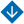Unconstrained Optimization of aTime-Varying Objective Function on a Discrete Time Scale
•About Advisory & Editorial Boards Aims & Scope Publication Ethics Review Process Archive Contact Information

#Unconstrained Optimization of aTime-Varying Objective Function on a Discrete Time Scale

Gusev, V.B. Unconstrained Optimization of aTime-Varying Objective Function on a Discrete Time Scale

Abstract. This paper develops an approximate method to optimize a time-varying objective function on a discrete time scale. The method should provide an admissible (controllable) error value. The conditions to be satisfied by the time scale, the objective function, and the environment’s parameters are established. The unconstrained optimization of a time-varying objective function that depends on the control vector components is considered on a discrete set of time instants. To find a solution, a discrete gradient constrained optimization method is proposed. Efficiency conditions for the gradient method are formulated. A lower bound on the solution error is obtained in terms of the time step, the rate of change of the objective function, and its first- and second-order derivatives with respect to the control vector components. The method is illustrated on a numerical example of an optimal controller design for a time-varying plant with a nonlinear objective function. According to the numerical experiments, the wide-range variations of the controller’s parameters have no significant effect on the qualitative behavior of the resulting trajectory. The method can be used to calculate an optimal control function for a system with a discrete-valued objective function.

Keywords: time-varying system, optimal controller, unconstrained optimization, lower bound on error.

PDF (English)